Next:Unified EntropyUp:Generalized Entropy Measures

Entropies of Order 1 and Degree s and Order r and Degree s

Sharma and Mittal (1975)  introduced and characterized two entropies called entropy of order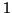and degree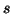and entropy of order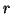and degreegiven by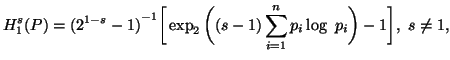(3.6)

and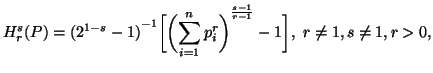(3.7)

respectively, for all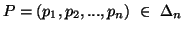, whereandare real parameters.

Sharma and Mittal's main motivation was to generalize the three entropies,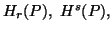and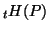. With this aim, they arrived at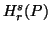reduces to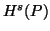and, when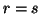and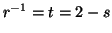, respectively.reduces to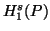and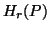when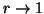and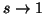respectively. Also,reduces to Shannon's entropy, H(P), when.

Thus, we see that the entropy of orderand degreecontain, either as a limiting or as a particular case, the Shannon's entropy, the entropy of order, the entropy of degree, the entropy of kind t, and the entropy of order 1 and degree.

We have presented in the previous subsections seven generalized entropies having one and/or two scalar parameters. A natural question arises at this stage, what kind of other generalized entropies exist in the literature. For a complete list we refer to section 3.6.2 (Taneja, 1989; 1990a) , .

21-06-2001
Inder Jeet Taneja
Departamento de Matemática - UFSC
88.040-900 Florianópolis, SC - Brazil